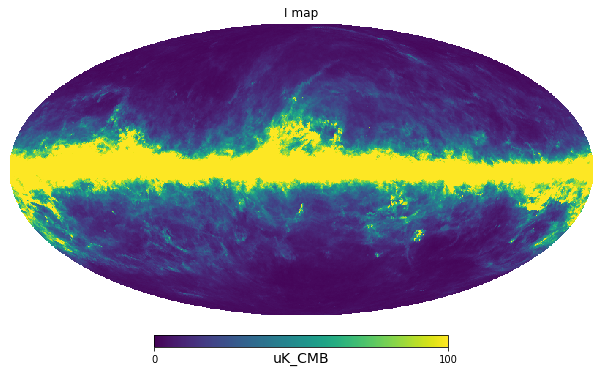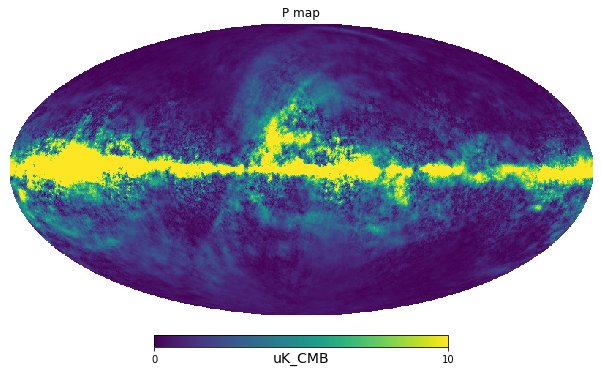# Getting started¶

The main interface to PySM is the pysm3.Sky class, the simplest way is to specify the required resolution as $$N_{side}$$ HEALPix parameter and the requested models as a list of strings, for example the simplest models for galactic dust and synchrotron ["d1", "s1"]

:

import pysm3
import pysm3.units as u
import healpy as hp
import numpy as np

:

import warnings
warnings.filterwarnings("ignore")

:

sky = pysm3.Sky(nside=128, preset_strings=["d1", "s1"])


PySM initializes the requested component objects (generally load the input templates maps with astropy.utils.data and cache them locally in ~/.astropy) and stores them in the components attribute (a list):

:

sky.components

:

[<pysm3.models.dust.ModifiedBlackBody at 0x7f5f0706d050>,
<pysm3.models.power_law.PowerLaw at 0x7f5f5463d250>]


PySM 3 uses astropy.units: http://docs.astropy.org/en/stable/units/ each input needs to have a unit attached to it, the unit just needs to be compatible, e.g. you can use either u.GHz or u.MHz.

:

map_100GHz = sky.get_emission(100 * u.GHz)


The output of the get_emission method is a 2D numpy array in the usual healpy convention, [I,Q,U], by default in $$\mu K_{RJ}$$:

:

map_100GHz[0, :3]

:

$[1.8686613,~1.9287539,~2.2371621] \; \mathrm{\mu K_{{RJ}}}$

Optionally convert to another unit using astropy.units

:

map_100GHz = map_100GHz.to(u.uK_CMB, equivalencies=u.cmb_equivalencies(100*u.GHz))

:

import matplotlib.pyplot as plt
%matplotlib inline

:

hp.mollview(map_100GHz, min=0, max=1e2, title="I map", unit=map_100GHz.unit):

hp.mollview(np.sqrt(map_100GHz**2 + map_100GHz**2), title="P map", min=0, max=1e1, unit=map_100GHz.unit)[ ]: